# Gardens

The garden has the square shape with circumference 124 m. Divide it into two rectangular gardens, one should circumference 10 meters more than the second. What size will have a gardens?

a =  31 m
b =  18 m
c =  31 m
d =  13 m

### Step-by-step explanation:

a = 124/4
c = a
2(a+b) = 10+2(c+d)
b+d = a

a = 124/4
c = a
2•(a+b) = 10+2•(c+d)
b+d = a

4a = 124
a-c = 0
2a+2b-2c-2d = 10
a-b-d = 0

a = 31
b = 18
c = 31
d = 13

Our linear equations calculator calculates it.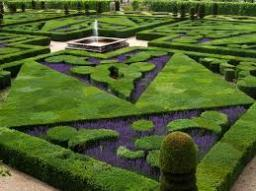Did you find an error or inaccuracy? Feel free to write us. Thank you!Tips to related online calculators
Do you have a linear equation or system of equations and looking for its solution? Or do you have a quadratic equation?

#### You need to know the following knowledge to solve this word math problem:

We encourage you to watch this tutorial video on this math problem:

## Related math problems and questions:

• Mr. ZucchiniMr. Zucchini had a rectangular garden whose perimeter is 28 meters. The garden's content area filled just four square beds, whose dimensions in meters are expressed in whole numbers. Determine what size could have a garden. Find all the possibilities and
• Garden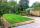The garden has a rectangular shape and has a circumference of 130 m and area 800.25 m2. Calculate the dimensions of the garden.
• Rectangular gardenThe perimeter of Peter's rectangular garden is 98 meters. The width of the garden is 60% shorter than its length. Find the dimensions of the rectangular garden in meters. Find the garden area in square meters.
• Rectangular garden 2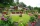A farmer bought 600 m of wire for the fence. He wants to use it to besiege a rectangular garden with a surface of 16875 m2. Calculate the size of the garden.
• Two gardens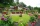The total area of the two gardens is 864 m2. The first garden is 60 m2 smaller than three times the second garden. What is the area of each garden?
• Three gardensIs it possible to divide a plot of land with an area of 920 m2 into three gardens in a ratio of 4: 2: 3 so that their areas are only whole multiples of square meters?
• SeedsThe field has a rectangular shape with dimensions of 128 m and 350 m. How many kg of seed are needed for sowing if the 1 m2 will consume 25 g of seeds?
• Unknown number 24I think the number: a - is the same as the square area that has the 12th circumference. What is this number? b - its half is 7 times bigger than its quarter. Is this the number?
• Rectangular gardenRectangular garden has a length of 48.7 m, a width of 6.3 meters shorter than the length. How much mesh should be bought for its fencing if the gate is 2.9 m long and the gate 1.1 m? What is the area of the garden?
• Fence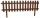How many meters of fencing it is required to purchase if our garden is rectangular measuring 20 m and 180 dm.
• GardenThe rectangular garden has dimensions of 27 m and 30 m. Peter and Katka split it in a ratio of 4:5. How many square meters did Katkin measure part of the garden?
• Two gardensThe flower garden has a square shape. The new garden has the shape of a rectangle, and one dimension is 8 m smaller, and the other is twice as large as in a square garden. What were the original garden dimensions and the new garden if both gardens' area i
• Fence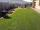The square garden has an area of 537 m2. How many meters is netting necessary to fence the garden?
• Fence of the garden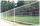The garden has dimensions of 5m and 400cm. How many meters meshes are needed for fencing the plot?
• GardenThe area of a square garden is 2/9 of triangle garden with sides 160 m, 100 m, and 100 m. How many meters of fencing need to fence a square garden?
• GardensThe area of the square garden is 3/4 of the area of the triangular garden with sides of 80 m, 50 m, 50 m. How many meters of the fence do we need to fence a square garden?
• Square into three rectangles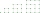Divide the square with a side length of 12 cm into three rectangles with have the same circumference so that these circumferences are as small as possible.